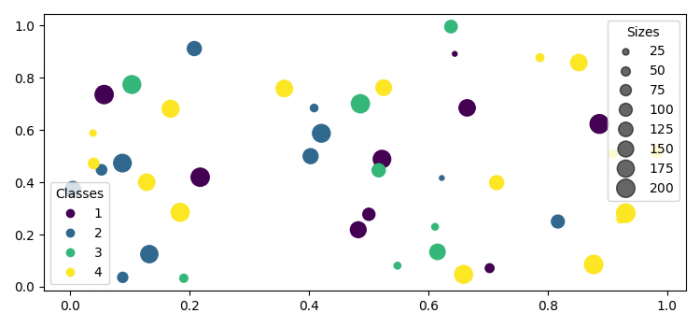# Automated legend creation in Matplotlib

To automate legend creation in Matplotlib, we can take the following steps −

• Set the figure size and adjust the padding between and around the subplots.
• Initialize a variable, N, for number of sample data.
• Create x, y, c and s data using numpy.
• Create a figure and a set of subplots using subplots() method.
• Plot x and y data points with different colors and sizes.
• Place a legend on the axes.
• Add an artist to the figure.
• Create legend handles and labels for a PathCollection.
• Again, place a legend on the axes for sizes.
• To display the figure, use show() method.

## Example

import matplotlib.pyplot as plt
import numpy as np

plt.rcParams["figure.figsize"] = [7.50, 3.50]
plt.rcParams["figure.autolayout"] = True

N = 45
x, y = np.random.rand(2, N)
c = np.random.randint(1, 5, size=N)
s = np.random.randint(10, 220, size=N)
fig, ax = plt.subplots()
scatter = ax.scatter(x, y, c=c, s=s)
legend1 = ax.legend(*scatter.legend_elements(), loc="lower left", title="Classes")
plt.show()